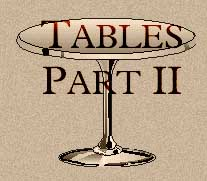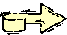If you are still looking for table furniture, this is not the page but I suggest not buying pine.

Tables Part II

## Options on the <TR> tag

The most useful option on the <TR> tag is to set the background color of a row. Using the table
```<TABLE border=0 cellpadding=10 cellspacing=0 bgcolor=white>
<TR>
<TH>upper left</TH>
</TR>
<TR>
<TD> this is row 1 column 1 </TD>
<TD> this is row 1 column 2 </TD>
<TD> this is row 1 column 3 </TD>
</TR>
<TR>
<TD> this is row 2 column 1 </TD>
<TD> this is row 2 column 2 </TD>
<TD> this is row 2 column 3 </TD>
</TR>
</TABLE>
```
By changing the first <TR> to <TR bgcolor="silver"> produces
row 1 column 1
this is
row 1 column 2
this is
row 1 column 3
row 2 column 1
this is
row 2 column 2
this is
row 2 column 3

## Options on the <TD> and <TH> tag

### Background Color

Like <TR>, the color on either the <TD> or <TH> tag can be changed. Changing the colors or the row headings to gray and the cell in the first row, second column to #FFFAB9
```<TABLE border =0 cellpadding=10 cellspacing=0 bgcolor=white>

<TR bgcolor="gray">
<TH>upper left</TH>
</TR>
<TR>
<TD> this is row 1 column 1 </TD>
<TD bgcolor="#FFFAB9"> this is row 1 column 2 </TD>
<TD> this is row 1 column 3 </TD>
</TR>
<TR>
<TD> this is row 2 column 1 </TD>
<TD  > this is row 2 column 2 </TD>
<TD> this is row 2 column 3 </TD>
</TR>
</TABLE>
```

produces
row header 1 this is row 1 column 1 this is row 1 column 2 this is row 1 column 3
row header 2 this is row 2 column 1 this is row 2 column 2 this is row 2 column 3

### Row and Column Span

Any cell can span multiple rows or columns. Give a 3 row by 4 column table:
```<TABLE BORDER>
<TR>
<TD>1</TD>
<TD>2</TD>
<TD>3</TD>
<TD>4</TD>
</TR>
<TR>
<TD>5</TD>
<TD>6</TD>
<TD>7</TD>
<TD>8</TD>
</TR>
<TR>
<TD>9</TD>
<TD>10</TD>
<TD>11</TD>
<TD>12</TD>
</TR>
</TABLE>
```

yields:
 1 2 3 4 5 6 7 8 9 10 11 12

I now wish to have cell 2 (row 1 column 2) to span two columns. This requires the elimination of the cell containing 3. I then change the <TD> entry for cell 2 to be <TD colspan=2>. The HTML now looks like:

```<TABLE BORDER>
<TR>
<TD>1</TD>
<TD colspan=2>2</TD>

<TD>4</TD>
</TR>
<TR>
<TD>5</TD>
<TD>6</TD>
<TD>7</TD>
<TD>8</TD>
</TR>
<TR>
<TD>9</TD>
<TD>10</TD>
<TD>11</TD>
<TD>12</TD>
</TR>
</TABLE>
```

The table is:
 1 2 4 5 6 7 8 9 10 11 12

One can also span rows by using ROWSPAN= within a <TD>. The following table has the cell containing 1 (row 1, column 1) spanning three rows. I will also need to eliminate one <TD> in row two and three (cells containing 5 and 9). This table looks like:
 1 2 4 6 7 8 10 11 12

Cells can span rows and columns. To have the cell containing 7 span 2 rows and two columns, the <TD> for cells 8, and 11 need to be deleted. The <TD> for cell 7 is changed to <TD rowspan=2 colspan=2>
 1 2 4 6 7 10

For better visual, the width of the above table was set at 70%.

### Alignment Within the Cells

As mentioned back in Tables (part 1), the <TH> automcatically centers text within cells. Default alignment of text (and images) within a cell can be overridden by specfying an ALIGN= for horizontal alignment and VALIGN= for vertical alignment. The values for alignment are TOP, CENTER and BOTTOM.

In the prior table, I want the 7 to be centered within the cell, the 1 appear in the center but at the top of the cell and the 6 to be centered.

The table:

```<TABLE BORDER width=70%>
<TR>
<TD rowspan=3>1</TD>
<TD colspan=2>2</TD>
<TD>4</TD>
</TR>
<TR>
<TD>6</TD>
<TD rowspan=2 colspan=2>7</TD>
</TR>
<TR>
<TD>10</TD>
</TR>
</TABLE>
```

has the following changes made (note the black text):

```<TABLE BORDER width=70%>
<TR>
<TD rowspan=3 align=center valign=top>1</TD>
<TD colspan=2>2</TD>
<TD>4</TD>
</TR>
<TR>
<TD align=center >6</TD>
<TD rowspan=2 colspan=2 align=center valign=center>7</TD>
</TR>
<TR>
<TD>10</TD>
</TR>
</TABLE>
```

 1 2 4 6 7 10

Onto Tables Part III